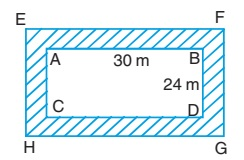# Perimeter and Area of Rectangle

The perimeter of rectangle ABCD as 2(AB + BC).

### Perimeter of Rectangle

If the perimeter is denoted by P, length as l and breadth as b. Then,

P = 2(l + b)

Example 1: Find the perimeter of a rectangle whose length is 20 cm and breadth is 8 cm.

Perimeter of rectangle = 2(20 + 8)

= 2 x 28 = 56 cm

Example 2: The perimeter of a rectangle is 46 cm, length is 15 cm, find the breadth of the rectangle.

46 = 2(15 + b)

23 = 15 + b

b = 8 cm

### Area of Rectangle

Area of rectangle = (length × breadth)

Area = l × b

Example 3: Find the area of a rectangle whose length is 5 cm and width is 3 cm.

Area = 5 x 3 = 15 cm2

Example 4: Find the length of a rectangle whose area is 400 cm2 and the width is 16 cm.

Area = Length x Width

400 = Length x 16

Length = 25 cm

### Rectangular Path

Example 5: A rectangular park of length 30 m and breadth 24 m is surrounded by a 4 m wide path. Find the area of the path.Let ABCD be the park and EFGH be the path (outer rectangle) surrounding it.

Length of rectangle EFGH = (30 + 4 + 4) m = 38 m

Breadth of rectangle EFGH = (24 + 4 + 4) m = 32 m

Area of path = area of rectangle EFGH - area of rectangle ABCD

= (38 × 32 - 30 × 24) m2

= (1216 - 720) m2

= 496 m2

Example 6: There are two rectangular paths in the middle of a park. Find the cost of paving the paths with concrete at the rate of Rs.15 per m2. It is given that AB = CD = 50 m, AD = BC = 40 m and EF = PQ = 2.5 m.Area of paths = Area of PQRS + Area of EFGH - area of square MLNO

= (40 × 2.5 + 50 × 2.5 - 2.5 × 2.5) m2

= 218.75 m2

So, cost of paving the concrete at the rate of Rs.15 per m2 = 218.75 × 15

= Rs.3281.25